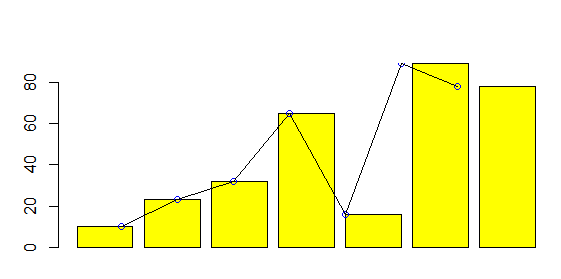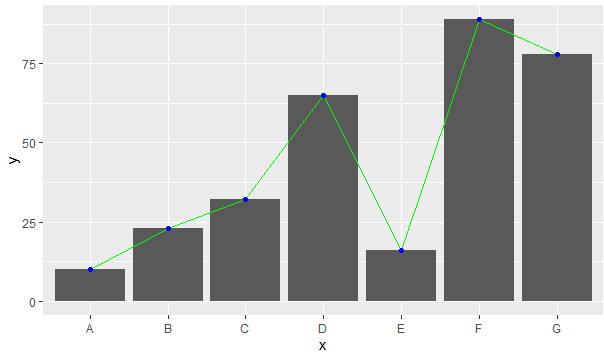Open in App
Not now

# Draw Multiple Graphs and Lines in Same Plot in R

• Last Updated : 06 Jun, 2021

A visualization can sometimes make more sense when multiple graphs and line plots are combined into one plot. In this article, we will discuss how we can do the same in the R programming language.

#### Method 1: Using base R

Base R supports certain methods that can be used to generate the desired plot. In this example plot, a scatter plot, a line plot, and a bar graph in the same frame for the same data.

barplot() function is used to produce a barplot with appropriate parameters.

Syntax:

barplot(H, xlab, ylab, main, names.arg, col)

Parameters:

• H: This parameter is a vector or matrix containing numeric values which are used in bar chart.
• xlab: This parameter is the label for x axis in bar chart.
• ylab: This parameter is the label for y axis in bar chart.
• main: This parameter is the title of the bar chart.
• names.arg: This parameter is a vector of names appearing under each bar in bar chart.
• col: This parameter is used to give colors to the bars in the graph.

points() function in R Language is used to add a group of points of specified shapes, size and color to an existing plot.

Syntax: points(x, y, cex, pch, col)

Parameters:
x, y: Vector of coordinates
cex: size of points
pch: shape of points
col: color of points

lines() function in R Language is used to add lines of different types, color, and width to an existing plot.

Syntax: lines(x, y, col, lwd, lty)

Parameters:
x, y: Vector of coordinates
col: Color of line
lwd: Width of line
lty: Type of line

The idea is simple and straightforward. Methods to add different visualizations just needs to be added to the code one by one, the plot will interpret each function and draw the plot accordingly.

Example:

## R

 `df<-``data.frame``(x = ``c``(``"A"``,``"B"``,``"C"``,``"D"``,``"E"``,``"F"``,``"G"``),``               ``y = ``c``(10,23,32,65,16,89,78))`` ` `barplot``(df\$y, xlab = df\$x, col = ``"yellow"``)``points``(df\$x, df\$y, type = ``"o"``,col = ``"blue"``)``lines``(df\$x, df\$y)`

Output:#### Method 2: Using ggplot

ggplot is a library supported by R which visualization easier. This again can be used to combine multiple graphs into one. A plot is generalized using ggplot() function and then all plots are added to the same plot using + sign.

Here, geom_bar() is used to draw the bar plot, geom_line() is used to draw the line chart and geom_point() is used for scatter plot.

Example:

## R

 `library``(ggplot2)`` ` `df<-``data.frame``(x = ``c``(``"A"``,``"B"``,``"C"``,``"D"``,``"E"``,``"F"``,``"G"``),``               ``y = ``c``(10,23,32,65,16,89,78),)`` ` `ggplot``(df, ``aes``(x, y, group = 1))+``geom_bar``(stat = ``"identity"``)+``geom_line``(color = ``"green"``)+``geom_point``(color = ``"blue"``)`

Output:My Personal Notes arrow_drop_up